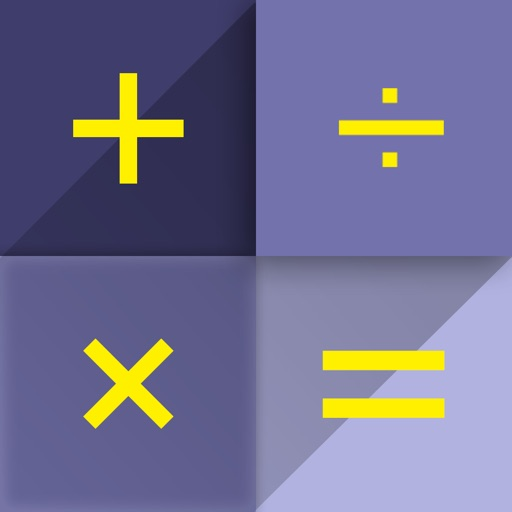## Calculator is a standard and scientific calculator application, perform calculations from basic to advanced# Calculator - Unit Converter

by TOH CO.,LTD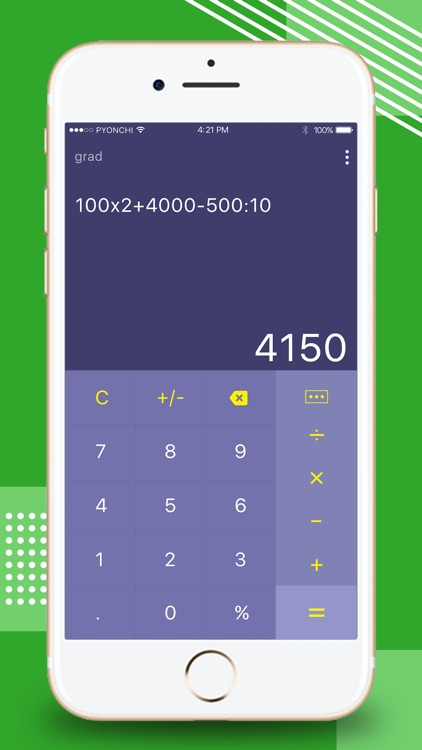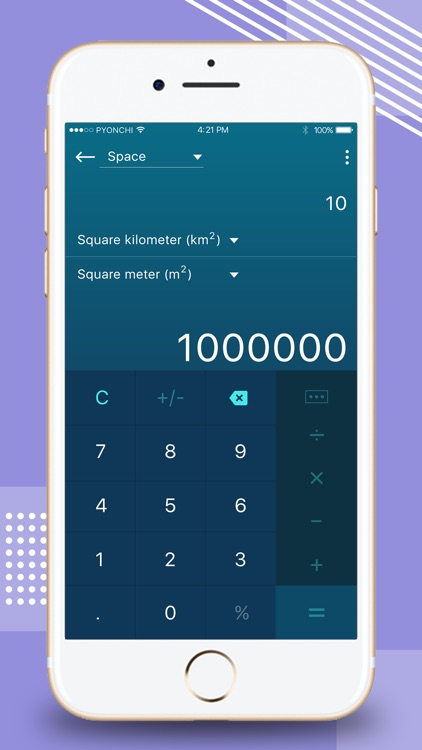Calculator is a standard and scientific calculator application, perform calculations from basic to advanced. The application also incorporates unit converter: Deg, Rad, Grad. This is a math calculation application simple and useful for everyone.### App Details

Version
1.0
Rating
(206)
Size
32Mb
Genre
Utilities Productivity
Last updated
March 16, 2018
Release date
March 16, 2018

### App Screenshots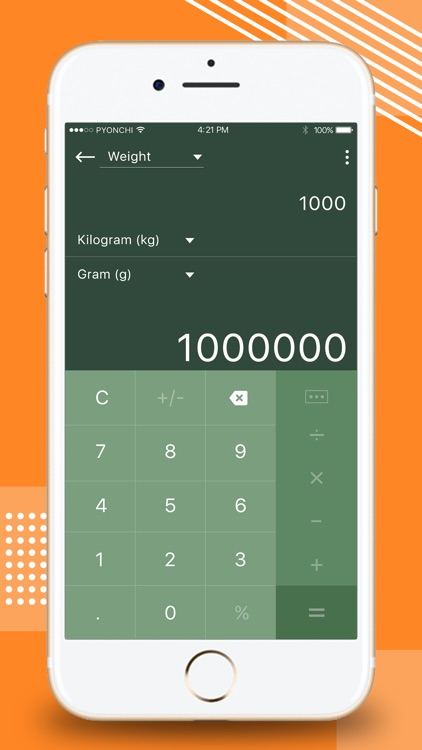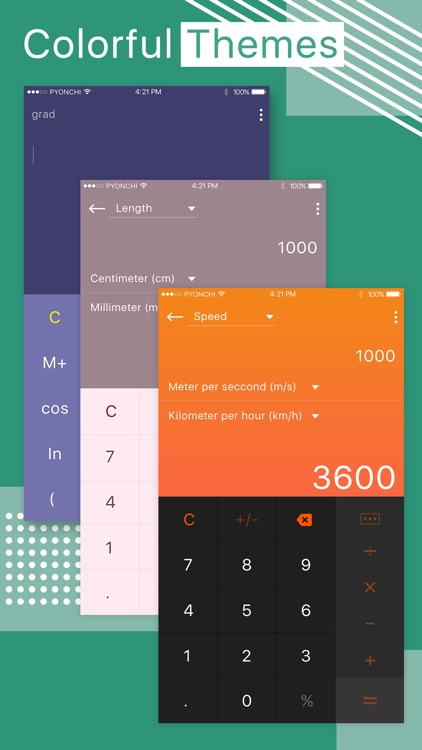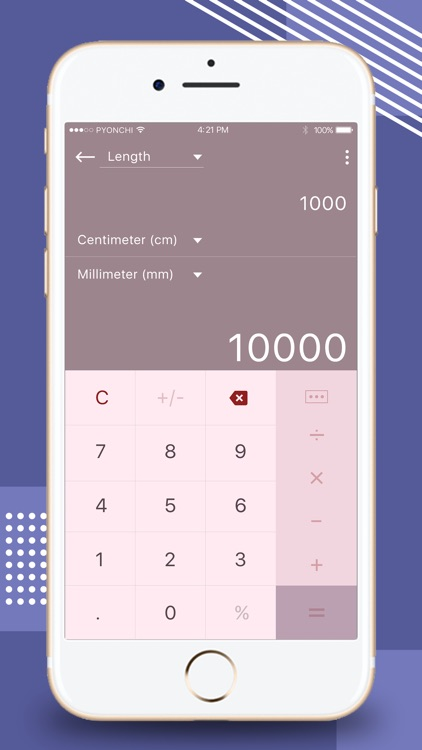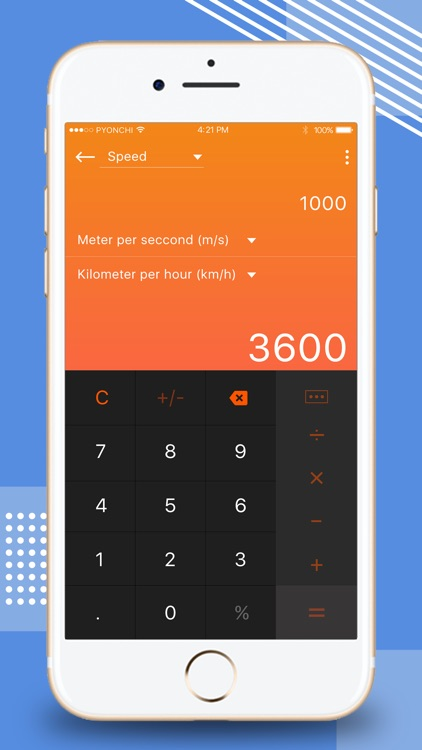### App Store Description

Calculator is a standard and scientific calculator application, perform calculations from basic to advanced. The application also incorporates unit converter: Deg, Rad, Grad. This is a math calculation application simple and useful for everyone.

The Calculator app helps you calculate the calculations from basic to advanced. Quickly perform simple mathematical operations such as plus, subtraction, multiplication, division, ... Accurately calculates complex calculations that contain exponentiation functions, logarithms, trigonometric functions, integrals,...
In particular, the Calculator app integrates measurement conversion functions such as length, speed, weight, space, temperature, etc. Unit converter allows you to easily switch units with just a few click.
Calculator - Unit Converter performs complex calculations and unit conversions as specialized calculators used in mathematics.
Features:
- Fast, accurate calculations from simple to complex.
- Simple calculation: plus, minus, multiplication, division, percent
- Complex calculations: decimal, power, logarithm, sine, cosine, tg, cosh, ...
- Functions (exponential functions, logarithms, trigonometric functions)
- Integral
- Estimation of factorial
- Linear equations, quadratic equations, polynomial equations.
- Matrices (matrix multiplications / matrixes with numbers, inverse matrix definitions)
- Convert units of measurement: length, speed, weight, space, temperature.
- Length converter: convert mm to cm (dm, m, km)
- Speed converter: convert m/s to km/h (mph, f/s)
- Temperature converter: convert oC to oF (K)
- Weight converter: convert g to kg (t, mg, Ib) and vice versa
- Simple design, easy to use, nice graphics